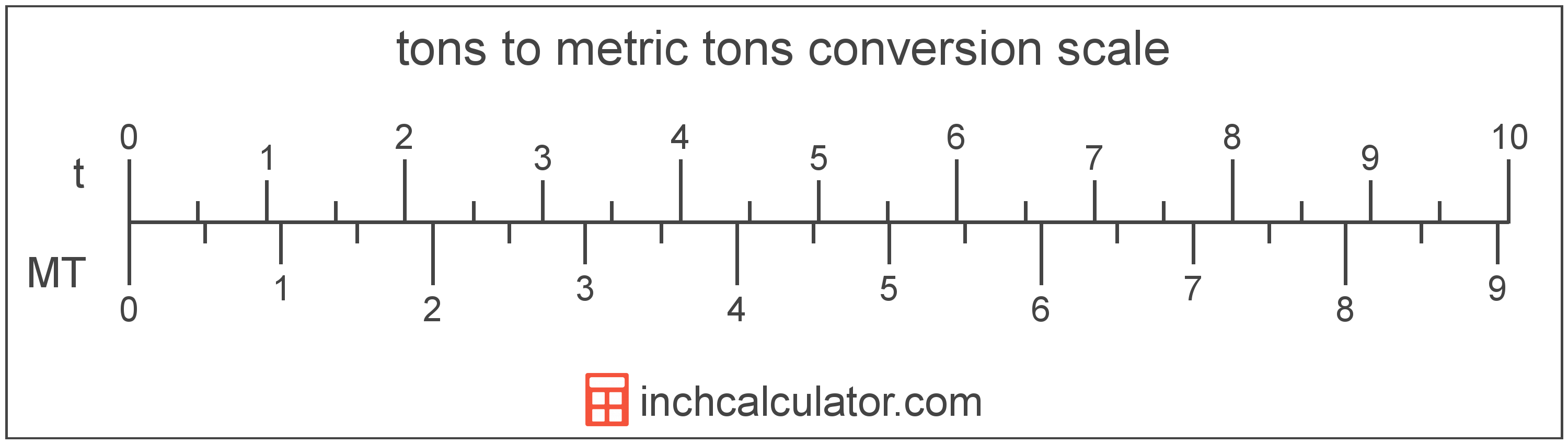Convert Tons to Metric Tons (Tonnes)

Enter the weight in tons below to get the value converted to metric tons.

Results in Metric Tons:1 t = 0.907185 t
Do you want to convert metric tons to tons?

How to Convert Tons to Metric TonsTo convert a ton measurement to a metric ton measurement, multiply the weight by the conversion ratio. One ton is equal to 0.907185 metric tons, so use this simple formula to convert:

metric tons = tons × 0.907185

The weight in metric tons is equal to the tons multiplied by 0.907185.

For example, here's how to convert 5 tons to metric tons using the formula above.
5 t = (5 × 0.907185) = 4.535924 t

Tons and metric tons are both units used to measure weight. Keep reading to learn more about each unit of measure.

Tons

One short ton, sometimes called a US ton, is equal to 2,000 pounds and is mostly used in the US as a unit of mass/weight. The short ton should not be confused with the long ton, which is used mostly in the United Kingdom, or the metric ton which is used in most other countries.

The ton is a US customary unit of weight. A ton is sometimes also referred to as a short ton. Tons can be abbreviated as t, for example 1 ton can be written as 1 t.

Metric Tons (Tonnes)

One tonne is equal to 1,000 kilograms or 2,204.6 pounds, and is used throughout the world as a unit of mass/weight. One tonne is also equal to 1 megagram. The metric ton should not be confused with short tons, used primarily in the US, or long tons, used primarily in the UK.

The metric ton is an SI accepted unit for weight for use with the metric system. A metric ton is sometimes also referred to as a tonne. Metric tons can be abbreviated as t, and are also sometimes abbreviated as T, Te, or MT. For example, 1 metric ton can be written as 1 t, 1 T, 1 Te, or 1 MT.

In the US the unit is referred to as the metric ton, while the rest of the world refers to it as the tonne. Most often it's still pronounced as tun, but it is sometimes pronounced tunny.

Ton Measurements and Equivalent Metric Ton Conversions

Common ton values converted to the equivalent metric ton value
Tons Metric Tons
1 t 0.907185 t
2 t 1.8144 t
3 t 2.7216 t
4 t 3.6287 t
5 t 4.5359 t
6 t 5.4431 t
7 t 6.3503 t
8 t 7.2575 t
9 t 8.1647 t
10 t 9.0718 t
11 t 9.979 t
12 t 10.89 t
13 t 11.79 t
14 t 12.7 t
15 t 13.61 t
16 t 14.51 t
17 t 15.42 t
18 t 16.33 t
19 t 17.24 t
20 t 18.14 t
21 t 19.05 t
22 t 19.96 t
23 t 20.87 t
24 t 21.77 t
25 t 22.68 t
26 t 23.59 t
27 t 24.49 t
28 t 25.4 t
29 t 26.31 t
30 t 27.22 t
31 t 28.12 t
32 t 29.03 t
33 t 29.94 t
34 t 30.84 t
35 t 31.75 t
36 t 32.66 t
37 t 33.57 t
38 t 34.47 t
39 t 35.38 t
40 t 36.29 t

More Ton Weight Conversions

US Customary & Imperial Units
Convert to Pounds
1 t is equal to 2,000 pounds
Convert to Ounces
1 t is equal to 32,000 ounces
Other Units
Convert to Long Tons
1 t is equal to 0.892857 long tons
Convert to Carats
1 t is equal to 4,535,923.7 carats
SI Units
Convert to Kilograms
1 t is equal to 907.18474 kilograms
Convert to Grams
1 t is equal to 907,184.7 grams
Convert to Milligrams
1 t is equal to 907,184,740 milligrams